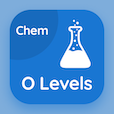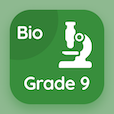Cambridge Online Courses (GCSE)

O Level Physics Quizzes

O Level Physics Quiz PDF - Complete

# Temperature Scales Quiz Questions Online p. 67

Learn Temperature Scales quiz questions and answers, temperature scales MCQ with answers PDF 67 to study O Level Physics course online. Temperature in Physics trivia questions, temperature scales Multiple Choice Questions (MCQ) for online college degrees. "Temperature Scales Quiz" PDF Book: melting and solidification, work and energy, speed, velocity and acceleration, scalar and vector, temperature scales test prep for ACT test prep classes.

"Boiling point of liquid hydrogen is equal to 20 K, which is equal to" MCQ PDF: −253 °c, −293 °c, 293 °c, and 253 °c for colleges offering online degree programs. Study temperature in physics questions and answers to improve problem solving skills for accelerated bachelors degree online.

## Quiz on Temperature Scales MCQs

MCQ: Boiling point of liquid hydrogen is equal to 20 K, which is equal to

−293 °C
−253 °C
293 °C
253 °C

MCQ: If two forces of 3 N acting on an object in opposite direction. The resultant force will be

6 N
3 N
9 N
Zero

MCQ: A car stops and then starts accelerating uniformly at rate of 3 m s-2, speed of car after 20 seconds is

40 m s-2
60 m s-2
100 m s-2
30 m s-2

MCQ: An object has Ep of 500 J with a mass of 25 kg and gravity of 10 N kg-1, height of object is

20 m
1250 m
2 m
125000 m

MCQ: Solid melts because the

intermolecular bonds get weak
thermal energy increases the rate of kinetic energy
thermal energy helps in breaking the intermolecular bonds between the particles
particles start vibrating more vigorously due to thermal energy

### More Quizzes from O Level Physics Course